×#### Thank you for registering.

One of our academic counsellors will contact you within 1 working day.

Click to Chat

1800-1023-196

+91-120-4616500

CART 0

• 0

MY CART (5)

Use Coupon: CART20 and get 20% off on all online Study Material

ITEM
DETAILS
MRP
DISCOUNT
FINAL PRICE
Total Price: Rs.

There are no items in this cart.
Continue Shopping```Relationship between A.M. and G.M.

Arithmetic Mean

Geometric Mean

How to calculate the Geometric mean of more than two numbers?

Relationship between A.M. and G.M.

Properties of relationship of A.M and G.M.

Arithmetic Mean

Arithmetic Mean is the average of two given numbers. If we have two numbers a and b, then we can include a number A in between these numbers so that the three numbers will form an arithmetic sequence like a, A, b.

Here the number A is the arithmetic mean of the numbers a and b.

According to the property of Arithmetic progression, we can say that-

A – a = b – A  that is, the common difference ‘d’ of the given AP.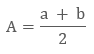This is generally used to find the missing number of the sequence between the two given numbers.The arithmetic mean serves as the balancing point of the two given numbers.

Example

What is the Arithmetic mean of 4 and 16 ?

Given

a = 4

b = 16

So the Arithmetic mean will be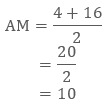Hence the AP is 10.

Can we insert two or more numbers between the two given numbers to form an Arithmetic progression?

Let A1 , A2 , A3 , …, An be n numbers between a and b such that  a, A1 , A2 , A3 , …,An , b is an A.P.

Here, b is the (n + 2) th term, i.e., b = a + [(n + 2) – 1] d = a + (n + 1) d.

This givesThus, n numbers between a and b are as follows: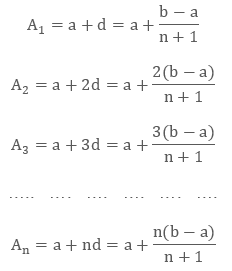Example

Insert 4 numbers between 3 and 18 such that the resulting sequence is an A.P.

Solution:

Let A1, A2, A3 and A4 be the four numbers between 3 and 18 such that

3, A1, A2, A3, A4, 18

is the sequence in A.P.

Here, a = 3, b = 18, n = 6.

Therefore,

18 = 3 + (6 –1) d

15 = 5d

d = 3

Thus   A 1 = a + d = 3 + 3 = 6;

A 2 = a + 2d = 3 + 2 × 3 = 9;

A 3 = a + 3d = 3 + 3 × 3 = 12;

A 4 = a + 4d = 3 + 4 × 3 = 15

Hence, four numbers between 3 and 18 are 6, 9, 12 and 15

Geometric Mean

Geometric mean is a special type of average of two numbers. If we have two numbers then we will multiply the numbers and then take the square root of it.

If a and b are the two numbers then the geometric mean will be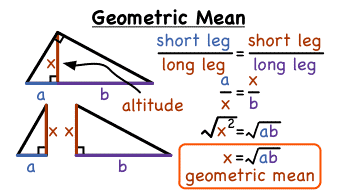Example

What is the geometric mean of 3 and 27?

Solution:

Given

a = 3 and b = 27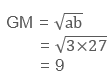Here we can see that the sequence 3, 9, 27 is a geometric progression.

How to calculate the Geometric mean of more than two numbers?

We can calculate the geometric mean of more than two numbers also. For calculating the geometric mean we have to multiply all the numbers and then take the nth root of that number.i.e. If we are multiplying two numbers, we are taking the square root, as we had taken in the above example.

If we will multiply three numbers then we will take the cube root. Likewise, if we are multiplying n number of terms then we will take the nth root of the number.

GM = n√ (a1 × a2 × ... × an)

Example

What is the Geometric Mean of 1, 4, 16, 64 and 256?

Solution:

Given

a1 = 1

a2 = 4

a3 =16

a4 = 64

a5 = 256

First we will multiply the given numbers

1 × 4 × 16 × 64 × 256 = 1048576

Then take the 5th root ( that is, the nth root where n = 4)

5√1048576 = 16

Geometric Mean = 5√ (1 × 4 × 16 × 64 × 256) = 16

Can we insert two or more numbers between the two given numbers to form a Geometric progression?

Let G 1, G2 ,…, G n be n numbers between positive numbers a and b such that

a, G 1, G 2, G 3,…, G n, b is a G.P.

Here, b is the (n + 2) th term, that is,Thus, n numbers between a and b is as follows: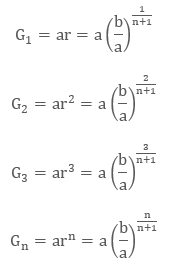Example

Insert 3 numbers between 1 and 81 such that the resulting sequence is a G.P.

Solution:

Let G 1, G2 and G3 ,be the three numbers between 1 and 81 such that

1, G 1, G2 , G3 , 81

the sequence is in G.P.

Here, a5 = 81

Hence r5 = 81 that is, r = 3

Thus, G 1 = ar = 1.3 = 3

G2 = ar2 = 1.32 = 9

G3 = ar3 = 1.33 = 27

Hence, three numbers between 1 and 81 are 3, 9 and 27 which in turn are making a Geometric sequence.

1, 3, 9, 27, 81

Relationship between A.M. and G.M.

As we have seen above the formula for the Arithmetic mean and the Geometric mean are as follows: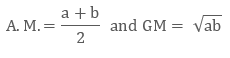where a and b are the two given positive numbers.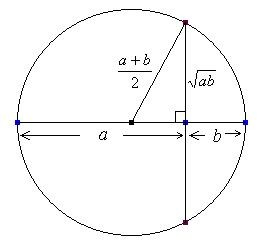Let  A and G be A.M. and G.M.

So,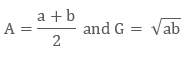Now let’s subtract the two of themThis shows that A ≥ G

Example

Find the two numbers, If Arithmetic mean and Geometric mean of two positive real numbers are 20 and 16, respectively.

Solution:

Given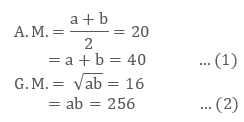Now we will put these values of a and b in

(a – b)2 = (a + b)2 – 4ab

(a – b)2 = (40)2 – 4(256)

= 1600 – 1024

= 576

a – b = ± 24 ( by taking the square root) …(3)

By solving (1) and (3), we get

a + b = 40

a – b = 24

a = 8, b = 32 or a = 32, b = 8

Properties of relationship of A.M and G.M.Property I: If the Arithmetic Mean and Geometric Mean of two positive numbers a and b are A and G respectively, then

A > G

As A – G > 0

Proof:

Let A and G be the Arithmetic Means and Geometric Means respectively of two positive numbers a and b.

Then,As, a and b are positive numbers, it is obvious that A > G when G = -√ab.

Now we have to show that A ≥ G when G = +√ab.

We have,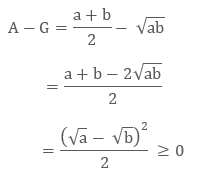Therefore, A - G ≥ 0 or, A ≥ G.

This proves that the Arithmetic Mean of two positive numbers can never be less than their Geometric Means.

Property II: If A be the Arithmetic Mean and G be the Geometric Mean between two positive numbers a and b, then the quadratic equation whose roots are a, b is

x2 - 2Ax + G2 = 0.

Proof:

As, A and G are the Arithmetic Mean and Geometric Mean respectively of two positive numbers a and b then, we haveThe equation having a, b as its roots is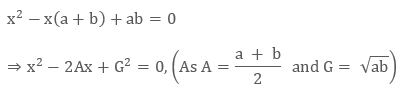Property III: If A is the Arithmetic Means and G be the Geometric Means between two positive numbers, and then the numbers are A ± √A2 – G2

Proof:

Since, A and G be the Arithmetic Means and Geometric Means respectively then, the equation having its roots as the given numbers isExample

Find two positive numbers whose Arithmetic Means increased by 2 than Geometric Means and their difference is 12.

Solution:

Let the two numbers be a and b. Then,

a-b = 12 ........................ (i)

Given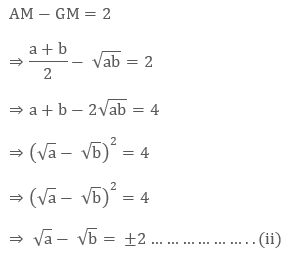Now, a - b = 12Solving (ii) and (iii), we get a = 16, b = 4

Hence, the required numbers are 16 and 4.

Example

If 34 and 16 are the Arithmetic Means and Geometric Means of two positive numbers respectively. Find the numbers.

Solution:

Let the two given positive numbers are a and b.

Given

Arithmetic Mean = 34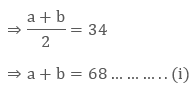Geometric Mean = 16As we know that

(a-b)2 = (a+b)2 – 4ab

⇒  (a-b)2 = (68)2 - 4 × 256 = 3600 ( from equation (i) and (ii))

⇒ a-b = 60............................... (iii)

After solving (i) and (iii), we get the numbers a = 64 and b = 4.

Hence, the required numbers are 64 and 4.

Relationship between A.M. and G.M.
```### Course Features

• 731 Video Lectures
• Revision Notes
• Previous Year Papers
• Mind Map
• Study Planner
• NCERT Solutions
• Discussion Forum
• Test paper with Video Solution